# How to Calculate and Solve for Total Load on Flexible Pipe | Irrigation Water Requirement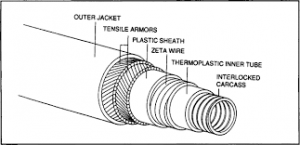The image above represents total load on flexible pipe.

To compute for total load on flexible pipe, three essential parameters are needed and these parameters are Load Co-efficient for Ditch Conduits (Cd), Unit Weight of Fill Material (w), Outside Diameter of the Conduit (Bc) and Width of Ditch at Top of Conduit (Bd).

The formula for calculating Total Load on Flexible Pipe:

Wc = CdwBcBd

Where:

Wc = Total Load on Flexible Pipe
Cd = Load Co-efficient for Ditch Conduits
w = Unit Weight of Fill Material
Bc = Outside Diameter of the Conduit
Bd = Width of Ditch at Top of Conduit

Let’s Solve an example;
Find the total load on flexible pipe when the load co-efficient for ditch conduits is 3, the unit weight of fill material is 5, the outside diameter of the conduit is 7 and width of ditch at top of conduit is 5.

This implies that;

Cd = Load Co-efficient for Ditch Conduits = 3
w = Unit Weight of Fill Material = 5
Bc = Outside Diameter of the Conduit = 7
Bd = Width of Ditch at Top of Conduit = 5

Wc = CdwBcBd
Wc = (3)(5)(7)(5)
Wc = 525

Therefore, the total load on flexible pipe is 525.

Calculating for the Load Co-efficient for Ditch Conduits when the Total Load on Flexible Pipe, the Unit Weight of Fill Material, Outside Diameter of the Conduit and the Width of Ditch at Top of Conduit is Given.

Cd = Wc / w x Bc x Bd

Where;

Cd = Load Co-efficient for Ditch Conduits
Wc = Total Load on Flexible Pipe
w = Unit Weight of Fill Material
Bc = Outside Diameter of the Conduit
Bd = Width of Ditch at Top of Conduit

Let’s Solve an example;
Find the load co-efficient for ditch conduits when the total load on flexible pipe is 40, the unit weight of fill material is 4, the outside diameter of the conduit is 6 and the Width of ditch at top of conduit is 2.

This implies that;

Wc = Total Load on Flexible Pipe = 40
w = Unit Weight of Fill Material = 4
Bc = Outside Diameter of the Conduit = 6
Bd = Width of Ditch at Top of Conduit = 2

Cd = Wc / w x Bc x Bd
Cd = 40 / 4 x 6 x 2
Cd = 40 / 48
Cd = 0.83

Therefore, the load co-efficient for ditch conduits is 0.83.

Calculating for the Unit Weight of Fill Material when the Total Load on Flexible Pipe, the Load Co-efficient for Ditch Conduits, Outside Diameter of the Conduit and the Width of Ditch at Top of Conduit is Given.

w = Wc / Cd x Bc x Bd

Where;

w = Unit Weight of Fill Material
Wc = Total Load on Flexible Pipe
Cd = Load Co-efficient for Ditch Conduits
Bc = Outside Diameter of the Conduit
Bd = Width of Ditch at Top of Conduit

Let’s Solve an example;
Find the unit weight of fill material when the total load on flexible pipe is 62, the load co-efficient for ditch conduits is 2, outside diameter of the conduit is 8 and the width of ditch at top of conduit is 4.

This implies that;

Wc = Total Load on Flexible Pipe = 62
Cd = Load Co-efficient for Ditch Conduits = 2
Bc = Outside Diameter of the Conduit = 8
Bd = Width of Ditch at Top of Conduit = 4

w = Wc / Cd x Bc x Bd
w = 62 / 2 x 8 x 4
w = 62 / 64
w = 0.96

Therefore, the unit weight of fill material is 0.96.

Calculating for the Outside Diameter of the Conduit when the Total Load on Flexible Pipe, the Load Co-efficient for Ditch Conduits, the Unit Weigth of Fill Material and the Width of Ditch at Top of Conduit is Given.

BcWc / Cd x w x Bd

Where;

Bc = Outside Diameter of the Conduit
Wc = Total Load on Flexible Pipe
Cd = Load Co-efficient for Ditch Conduits
w = Unit Weight of Fill Material
Bd = Width of Ditch at Top of Conduit

Let’s solve an example;
Find the outside diameter of the conduit when the total load on flexible pipe is 30, the load co-efficient for ditch conduits is 14, the unit weight of fill material is 10, the width of ditch at top of conduit is 8.

This implies that;

Wc = Total Load on Flexible Pipe = 30
Cd = Load Co-efficient for Ditch Conduits = 14
w = Unit Weight of Fill Material = 10
Bd = Width of Ditch at Top of Conduit = 8

BcWc / Cd x w x Bd
Bc30 / 14 x 10 x 8
Bc30 / 1120
Bc = 0.026

Therefore, the outside diameter of the conduit is 0.026.

Calculating for the Width of Ditch at Top of Conduit when the Total Load on Flexible Pipe, Load Co-efficient for Ditch Conduits, the Unit Weight of Fill Material and Outside Diameter of the Conduit is Given.

BdWc / Cd x w x Bc

Where;

Bd = Width of Ditch at Top of Conduit
Wc = Total Load on Flexible Pipe
Cd = Load Co-efficient for Ditch Conduits
w = Unit Weight of Fill Material
Bc = Outside Diameter of the Conduit

Let’s Solve an example;
Find the width of ditch at top of conduit when the total load on flexible pipe is 72, the load co-efficient for ditch conduits is 3, the unit weight of fill material is 4 and the outside diameter of the conduit is 10

This implies that;

Wc = Total Load on Flexible Pipe = 72
Cd = Load Co-efficient for Ditch Conduits = 3
w = Unit Weight of Fill Material = 4
Bc = Outside Diameter of the Conduit = 10

BdWc / Cd x w x Bc
Bd72 / 3 x 4 x 10
Bd72 / 120
Bd = 0.6

Therefore, the width of ditch at top of conduit is 0.6.

Nickzom Calculator – The Calculator Encyclopedia is capable of calculating the total load on flexible pipe.

To get the answer and workings of the total load on flexible pipe using the Nickzom Calculator – The Calculator Encyclopedia. First, you need to obtain the app.

You can get this app via any of these means:

To get access to the professional version via web, you need to register and subscribe for NGN 2,000 per annum to have utter access to all functionalities.
You can also try the demo version via https://www.nickzom.org/calculator

Apple (Paid) – https://itunes.apple.com/us/app/nickzom-calculator/id1331162702?mt=8
Once, you have obtained the calculator encyclopedia app, proceed to the Calculator Map, then click on Agricultural under Engineering.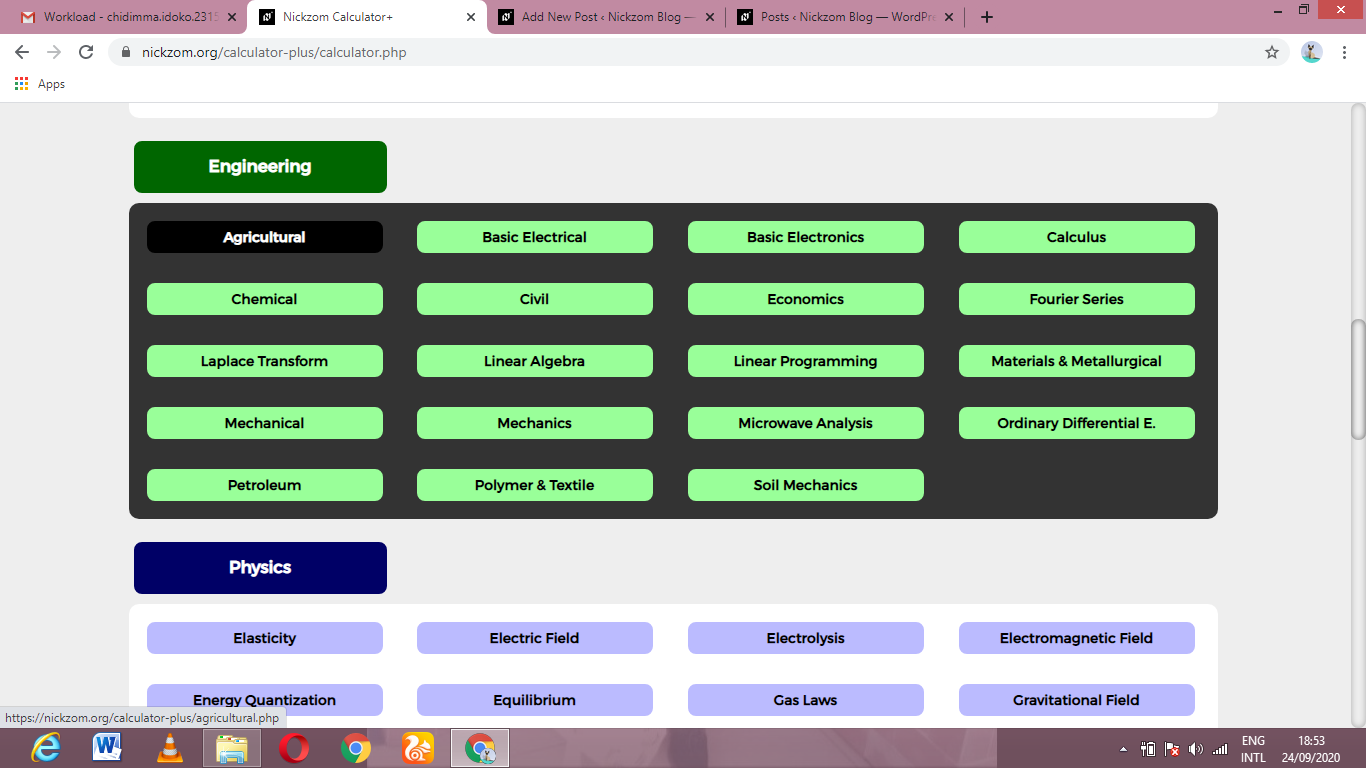Now, Click on Irrigation Water Requirement under AgriculturalNow, Click on Total Load on Flexible Pipe under Irrigation Water RequirementThe screenshot below displays the page or activity to enter your values, to get the answer for the total load on flexible pipe according to the respective parameters which is the Load Co-efficient for Ditch Conduits (Cd), Unit Weight of Fill Material (w), Outside Diameter of the Conduit (Bc) and Width of Ditch at Top of Conduit (Bd).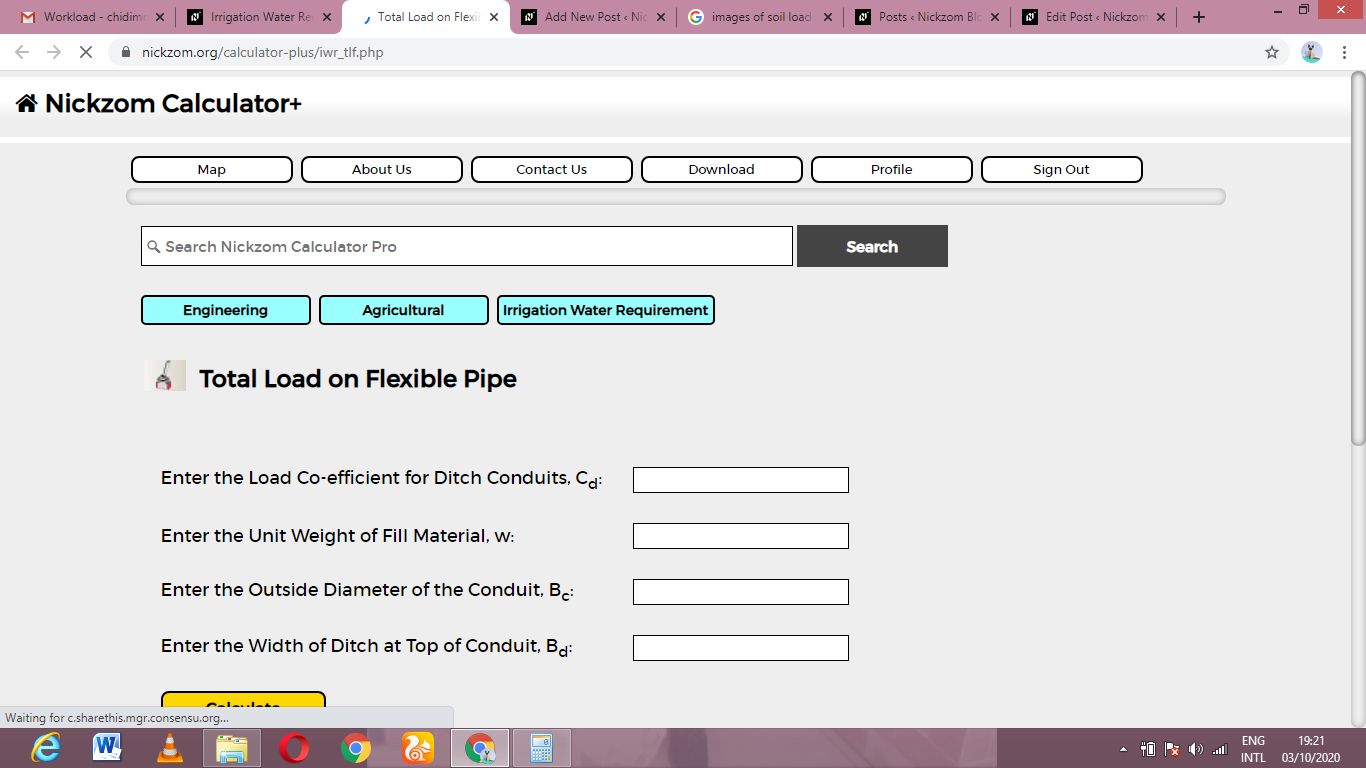Now, enter the values appropriately and accordingly for the parameters as required by the Load Co-efficient for Ditch Conduits (Cd) is 3, Unit Weight of Fill Material (w) is 5, Outside Diameter of the Conduit (Bc) is 7 and Width of Ditch at Top of Conduit (Bd) is 5.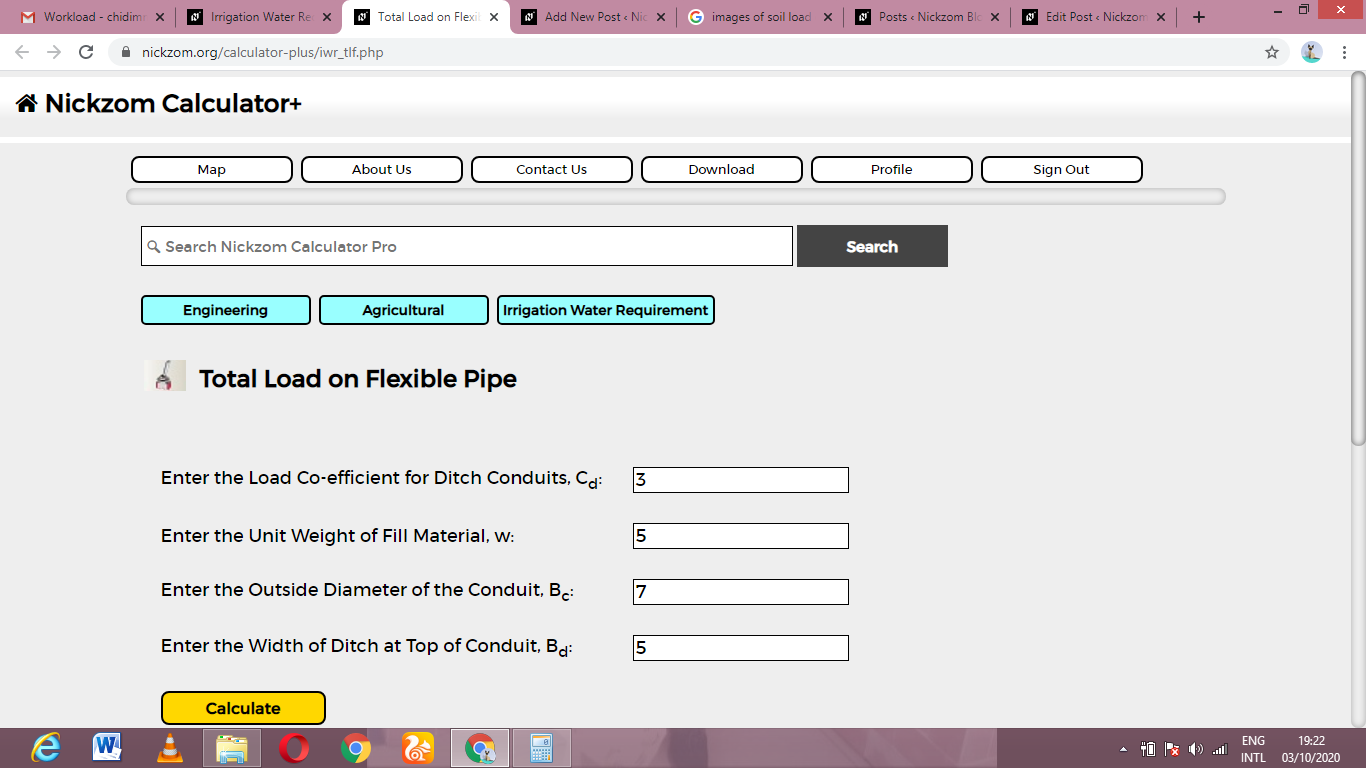Finally, Click on Calculate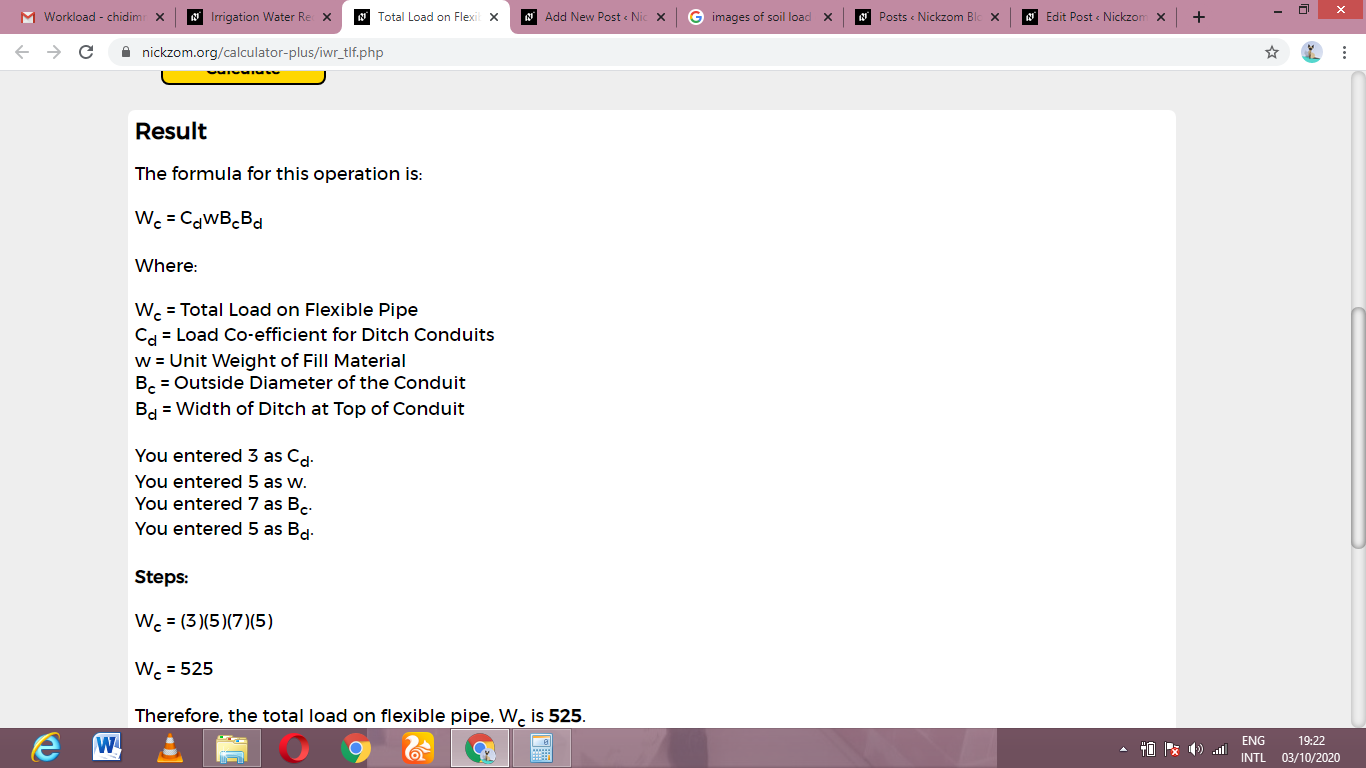As you can see from the screenshot above, Nickzom Calculator– The Calculator Encyclopedia solves for the total load on flexible pipe and presents the formula, workings and steps too.